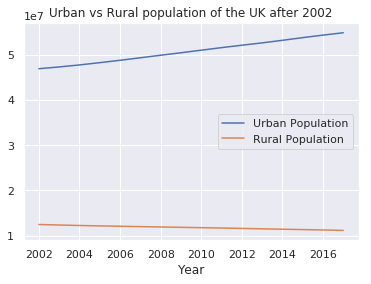## Blog for Coders# Introduction¶

Welcome to the first example of Data Science for Everyone. The following example will be about introduction to data visualization with Python and plotting line charts.

In the introductory examples, we'll use Google's CoLab which provides all the libraries and the environment we'll need. You can always modify the given code snippets by clicking the play button on the left side of each code cell after modifying them.

First, import pandas library, data structure and processing library for Python. By using as keyword, we can use pandas with pd alias.

In [ ]:
import pandas as pd


We'll use a subset of World bank's UK data which includes:

• Urban population,
• Rural population
• GDP per capita (constant LCU)
• GDP (constant LCU)
• CO2 emissions (metric tons per capita)
• Electric power consumption (kWh per capita)

Download the data formatted as CSV using pandas' read_csv function and assign it to variable data.

data variable is a DataFrame which is a tabular data format of pandas. We can quickly overview the first 5 rows of data frames using head function.

In [ ]:
# Read the data over a network.

# Display the first 5 rows of the data

Out[ ]:
Year Urban population Rural population GDP per capita (constant LCU) GDP (constant LCU) CO2 emissions (metric tons per capita) Electric power consumption (kWh per capita)
0 1960 41104656 11295344 8948.839808 4.689190e+11 11.150759 2412.137405
1 1961 41381472 11418528 9109.605488 4.809870e+11 11.154139 2553.693182
2 1962 41661202 11588798 9152.575522 4.873750e+11 11.142928 2780.657277
3 1963 41900114 11749886 9443.734631 5.066560e+11 11.254853 3002.087605
4 1964 42098400 11901600 9855.243363 5.321830e+11 11.265839 3127.462963

To have a quick analysis over the columns, we can use pandas' describe() function which gives the basic statistics including min, max, average, standard deviation and quartiles for each column.

In [ ]:
data.describe()

Out[ ]:
Year Urban population Rural population GDP per capita (constant LCU) GDP (constant LCU) CO2 emissions (metric tons per capita) Electric power consumption (kWh per capita)
count 58.000000 5.800000e+01 5.800000e+01 58.000000 5.800000e+01 55.000000 55.000000
mean 1988.500000 4.585411e+07 1.218020e+07 18046.455670 1.065813e+12 9.841130 4908.429642
std 16.886879 3.538235e+06 4.422552e+05 6093.622777 4.157194e+11 1.308239 1019.614068
min 1960.000000 4.110466e+07 1.112955e+07 8948.839808 4.689190e+11 6.497440 2412.137405
25% 1974.250000 4.361200e+07 1.191889e+07 12929.046842 7.269408e+11 9.050457 4486.378521
50% 1988.500000 4.458450e+07 1.227413e+07 18191.241670 1.040145e+12 9.870809 5082.439806
75% 2002.750000 4.722549e+07 1.255638e+07 24508.062405 1.460185e+12 10.988541 5671.992229
max 2017.000000 5.489374e+07 1.283936e+07 27629.745670 1.824210e+12 11.823036 6270.984059

### Access rows and columns of the data¶

We can access data frames' columns like data['Urban population']. It will gives us the entire column of the data.

To access data row by row, we can use data.iloc, where 0 is the row number. Notice that row indexes starts from 0.

In [ ]:
# Print the first row
data.iloc

Out[ ]:
Year                                           1.960000e+03
Urban population                               4.110466e+07
Rural population                               1.129534e+07
GDP per capita (constant LCU)                  8.948840e+03
GDP (constant LCU)                             4.689190e+11
CO2 emissions (metric tons per capita)         1.115076e+01
Electric power consumption (kWh per capita)    2.412137e+03
Name: 0, dtype: float64

# Visualizing¶

Now that we have data, we need to visualize it to have deeper understanding. So, let's begin visualization!

We'll use Matplotlib library for data visualization. Like pd, we create plt alias for matplotlib library. Then, to have nice looking figures, we import seaborn library (as sns) and set our chart styles using sns.set().

Since everything's imported for the plotting, we can begin the real work :) Using matplotlib's plot(x,y) function, we plot urban population over years. To do that, we select Year column as data['Years'] and Urban Population as data['Urban population']. We can show the plot using show() function.

In [ ]:
# import matplotlib library to use as plt
import matplotlib.pyplot as plt

# Imprt and set seaborn styles for nice chart styles
import seaborn as sns
sns.set()

# Plot the data: plt.plot(x-axis, y-axis)
plt.plot(data['Year'], data['Urban population'])

# Show the previously plotted data
plt.show()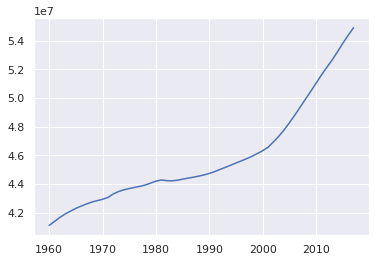Visual data is quicker to understand and easy to remember, thanks to Picture Superiority Effect. By observing the chart above for a few seconds, we can easily say urban population of UK has been a increasing with a constant rate after 2000. We wouldn't have this insight just by looking the numbers.

But, a chart without axis labels and title isn't useful. Let's add this chart a title and labels for both axis so that our plot become much more meaningful, even for complete outsiders.

Let's plot all other metrics in the same way.

In [ ]:
plt.plot(data['Year'],data['Urban population'])

# setting x & y axises labels
plt.xlabel('Year')
plt.ylabel('Urban population')

# setting plot title
plt.title('UK Urban Population')

# showing final plot
plt.show()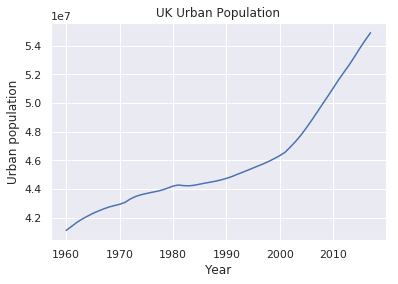### Changing line colour¶

We can change the line color by parameter c. You can use different colors like

• b: blue
• g: green
• r: red
• c: cyan
• m: magenta
• y: yellow
• k: black
• w: white
In [ ]:
# Plot the GDP per capita with red color using c='r'
plt.plot(data['Year'],data['GDP per capita (constant LCU)'],c='r')

# setting axis labels and plot title
plt.xlabel('Year')
plt.ylabel('GDP per capita')
plt.title('GDP per capita for the UK')

# showing plot
plt.show()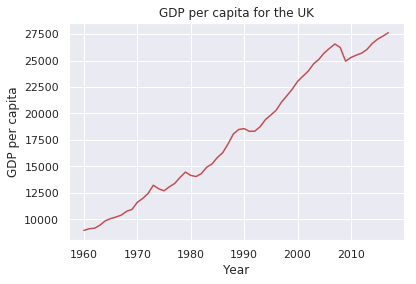## Plotting multiple data into one chart¶

How about plotting two relative data into one plot? We can do so by calling the plot function, twice 😊

As you can see below, matplotlib automatically chooses different colors for each plot function.

In [ ]:
# First plot Urban population
plt.plot(data['Year'],data['Urban population'])
# Then plot Rural population on the same figure
plt.plot(data['Year'],data['Rural population'])

# Set x axis label and title
plt.xlabel('Year')
plt.title('Urban & Rural population comparison of the UK')

# Show the final results
plt.show()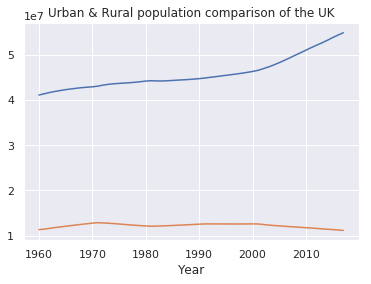Without prioir knowledge, we can't say which line represents urban population or rural population. So we need to add legend to our chart. To do so, we can call plt.legend() and it places a nice legend inside our chart.

In [ ]:
# First plot Urban population
plt.plot(data['Year'],data['Urban population'])
# Then plot Rural population on the same figure
plt.plot(data['Year'],data['Rural population'])

# Set x axis label and title
plt.xlabel('Year')
plt.title('Urban & Rural population comparison of the UK')

# Adding legend to current plot
plt.legend()

# Show the final results
plt.show()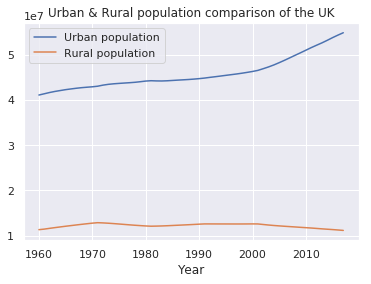## Filtering data¶

To be able to focus on the data after 2002, we may need to filter our data. To be able to do so, we need to select only the rows with Year column is greater or equal to 2000. We can create a mask to define which rows we are interested in and which we are not.

To select rows which Year column is greater or equal to 2002, we can use data['Year'] >= 2002. This will give us a mask indicating which rows before 2002 and which rows after 2002. Let's create a variable mask and mask the whole data. Then, we can plot the same chart using the code snippet above.

Note that legend is positioned according to chart's content.

In [ ]:
# Creating a mask for year >= 2002

# Applying mask to the data and assign filtered data to a new variable